A vector is a quantity that has magnitude and direction. For example, a velocity of 70 MPH North is a vector quantity because it has a magnitude of 70MPH and a direction of North. The most common vector notations are a bold-faced lower case letter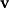and a letter with an overhead arrow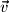. In contrast to a vector, a quantity with only magnitude is called a scalar. A vector is distinctly different than a point; however, students often confuse the two since both can be written as a grouping of numbers. The following shows the definition of a 3D vectorand a 3D point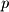: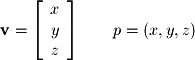The main distinction between a vector and a point is that two points are equivalent only if they share exactly the same coordinates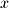,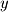, and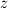. Two vectors are equivalent if they have the same magnitude and direction. A vector can be visualized graphically as a directed line segment with a starting point and an end point. If the starting point for a vector is not specified it is generally assumed that the starting point is the origin (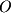). In Figure 1-8, the vector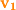has its tail at the origin () and its head at the end point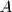. Although vector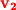starts at point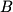and ends at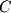, it is equivalent to vectorsince they both have the same magnitude and direction.
Object could not be loaded.
Figure 1-8.
VectorsandSince we will often refer to a vector with arbitrary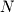dimensions (rather than with a specific  number of dimensions like in the 3 dimensional vector above), it is useful now to point out the formal notation for a vector of length: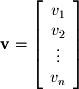The "dots" are ellipses and represent all the values that are assumed to exist between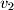and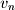. It is common in mathematics to write a finite series as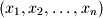because the first value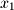specifies the initial subscript. The second value specifies the direction of the series which in this example is increasing. For example if the second subscript had been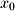as in the case of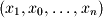the sequence would have been decreasing. The final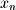specifies that the sequence terminates at the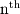value and thus is a finite sequence.

Finally, the other vector you will encounter throughout this text is the zero vector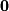: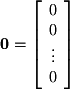The zero vector is written as a bold-facedor a zero with an arrow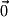. You should be aware when you see it that the zero vector is not the same as a single scalar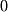.